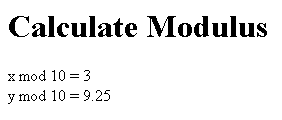Ads

Tutorials

# JSP Modulus

JSP Modulus is used to determine the modulus of number in jsp. Modulus is the remainder of division operation.

JSP Modulus is used to determine the modulus of number in jsp. Modulus is the remainder of division operation.

# JSP Modulus

JSP Modulus is used to determine the modulus of number in jsp. Modulus is the remainder of division operation.

Understand with Example

In this section, you will learn how to determine the modulus of number in jsp. As you already know that modulus is the remainder of division operation. You can see in the given example that we have create two variables i.e. x of integer type and y of float type and assign values to them. Then, we have used the modulus operator, % that will return the remainder of a division operation after dividing the number by 10.

Here is the code of modulus.jsp:



Calculate Modulus

<% int x = 33; double y = 99.25; out.println("x mod 10 = " + x % 10+"
"); out.println("y mod 10 = " + y % 10); %>

Output will be displayed as:Download Source Code:

Ads

Ads